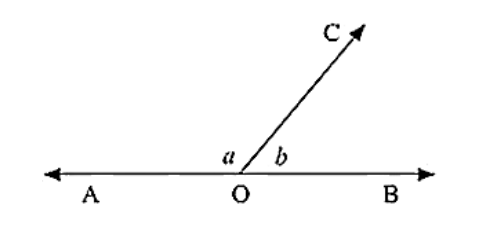# In the below figure, ∠AOC and ∠BOC form a linear pair. If a - 2b = 30°, find a and b?`
Question:

In the below figure, ∠AOC and ∠BOC form a linear pair. If a - 2b = 30°, find a and b?Solution:

Given that,

∠AOC and ∠BOC form a linear pair

If a - b = 30

∠AOC = a°, ∠BOC = b°

Therefore, a + b = 180 ... (1)

Given a - 2b = 30 ... (2)

By subtracting (1) and (2)

a + b - a + 2b = 180 - 30

3b = 150

b = 150/3

b = 50

Since a - 2b = 30

a - 2(50) = 30

a = 30 + 100

a = 130

Hence, the values of a and b are 130° and 50° respectively.